# http www comp nus edu sgcs 1010 UNIT

• Slides: 42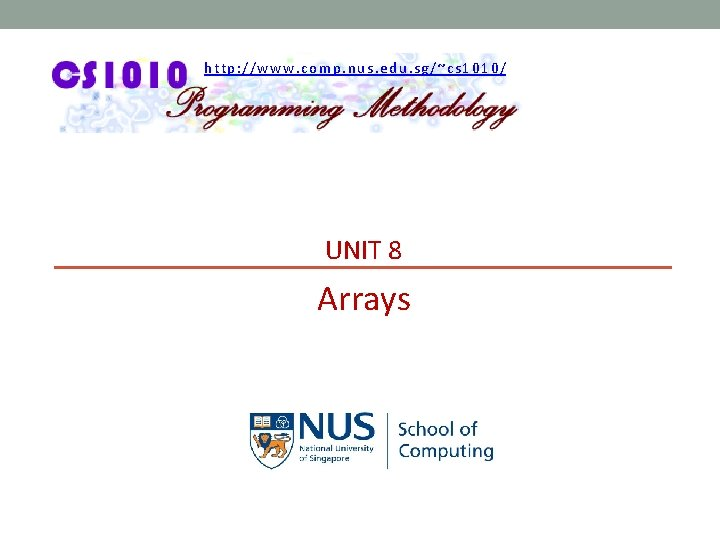http: //www. comp. nus. edu. sg/~cs 1010/ UNIT 8 ArraysAaron Tan, NUS Arrays UNIT 8 - 2 UNIT 8: Arrays Objectives: § Understand the concept and application of arrays § Problem solving using arrays Reference: § Chapter 7: Array Pointers § Sections 7. 1 – 7. 5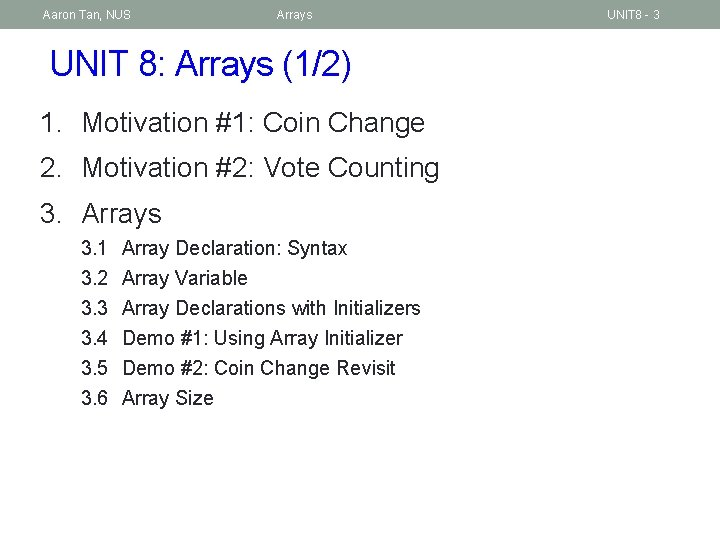Aaron Tan, NUS Arrays UNIT 8: Arrays (1/2) 1. Motivation #1: Coin Change 2. Motivation #2: Vote Counting 3. Arrays 3. 1 3. 2 3. 3 3. 4 3. 5 3. 6 Array Declaration: Syntax Array Variable Array Declarations with Initializers Demo #1: Using Array Initializer Demo #2: Coin Change Revisit Array Size UNIT 8 - 3Aaron Tan, NUS Arrays UNIT 8: Arrays (2/2) 4. Arrays and Pointers 5. Array Assignment 6. Array Parameters in Functions 7. Passing Array Arguments 8. Standard I/O Functions for Arrays 9. Modifying Array Arguments 10. Exercise: Up-slopes UNIT 8 - 4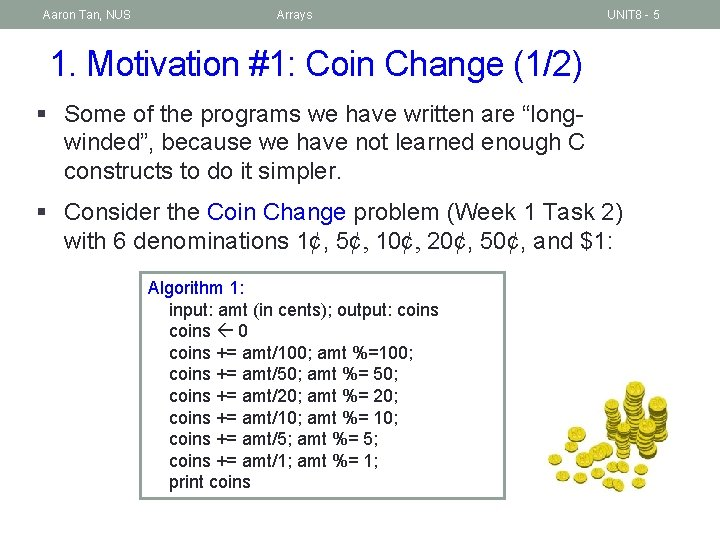Aaron Tan, NUS Arrays UNIT 8 - 5 1. Motivation #1: Coin Change (1/2) § Some of the programs we have written are “longwinded”, because we have not learned enough C constructs to do it simpler. § Consider the Coin Change problem (Week 1 Task 2) with 6 denominations 1¢, 5¢, 10¢, 20¢, 50¢, and \$1: Algorithm 1: input: amt (in cents); output: coins 0 coins += amt/100; amt %=100; coins += amt/50; amt %= 50; coins += amt/20; amt %= 20; coins += amt/10; amt %= 10; coins += amt/5; amt %= 5; coins += amt/1; amt %= 1; print coinsAaron Tan, NUS Arrays Unit 8 - 6 1. Motivation #1: Coin Change (2/2) int minimum. Coins(int amt) { int coins = 0; coins += amt/100; amt %= 100; coins += amt/50; amt %= 50; coins += amt/20; amt %= 20; coins += amt/10; amt %= 10; coins += amt/5; amt %= 5; coins += amt/1; // amt %= 1; // return coins; } Unit 8_Coin. Change. c § Can we do better? retained for regularity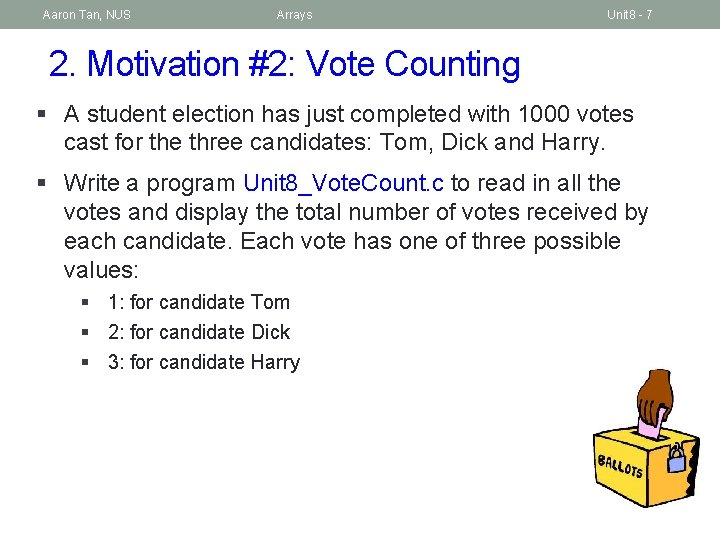Aaron Tan, NUS Arrays Unit 8 - 7 2. Motivation #2: Vote Counting § A student election has just completed with 1000 votes cast for the three candidates: Tom, Dick and Harry. § Write a program Unit 8_Vote. Count. c to read in all the votes and display the total number of votes received by each candidate. Each vote has one of three possible values: § 1: for candidate Tom § 2: for candidate Dick § 3: for candidate HarryAaron Tan, NUS Arrays Unit 8 - 8 2. Motivation #2: Votes for 3 candidates #include <stdio. h> #define NUM_VOTES 1000 Unit 8_Vote. Count. c // number of votes int main(void) { int i, vote, tom = 0, dick = 0, harry = 0; printf("Enter votes: n"); for (i = 0; i < NUM_VOTES; i++) { scanf("%d", &vote); What if there were switch (vote) { case 1: tom++; break; instead of 3 candidates? case 2: dick++; break; case 3: harry++; break; } } printf("Tom: %d; Dick: %d; Harry: %dn", tom, dick, harry); return 0; 30 }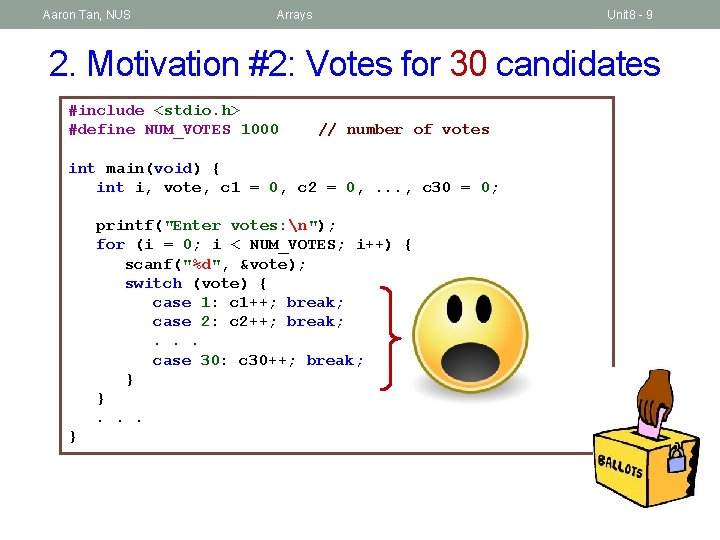Aaron Tan, NUS Arrays Unit 8 - 9 2. Motivation #2: Votes for 30 candidates #include <stdio. h> #define NUM_VOTES 1000 // number of votes int main(void) { int i, vote, c 1 = 0, c 2 = 0, . . . , c 30 = 0; printf("Enter votes: n"); for (i = 0; i < NUM_VOTES; i++) { scanf("%d", &vote); switch (vote) { case 1: c 1++; break; case 2: c 2++; break; . . . case 30: c 30++; break; } }. . . }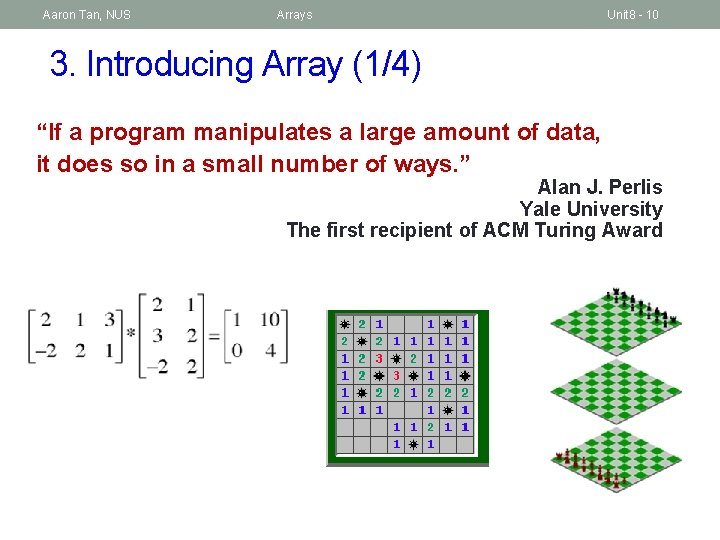Aaron Tan, NUS Arrays Unit 8 - 10 3. Introducing Array (1/4) “If a program manipulates a large amount of data, it does so in a small number of ways. ” Alan J. Perlis Yale University The first recipient of ACM Turing Award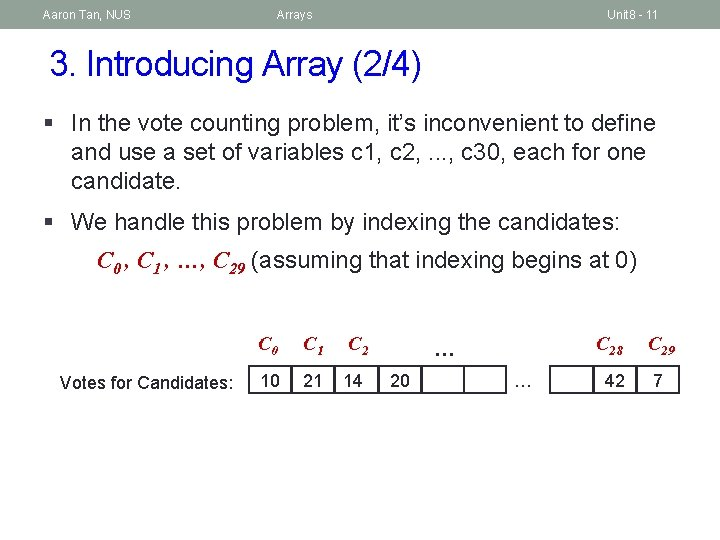Aaron Tan, NUS Arrays Unit 8 - 11 3. Introducing Array (2/4) § In the vote counting problem, it’s inconvenient to define and use a set of variables c 1, c 2, . . . , c 30, each for one candidate. § We handle this problem by indexing the candidates: C 0 , C 1 , …, C 29 (assuming that indexing begins at 0) Votes for Candidates: C 0 C 1 10 21 C 2 14 … 20 … C 28 C 29 42 7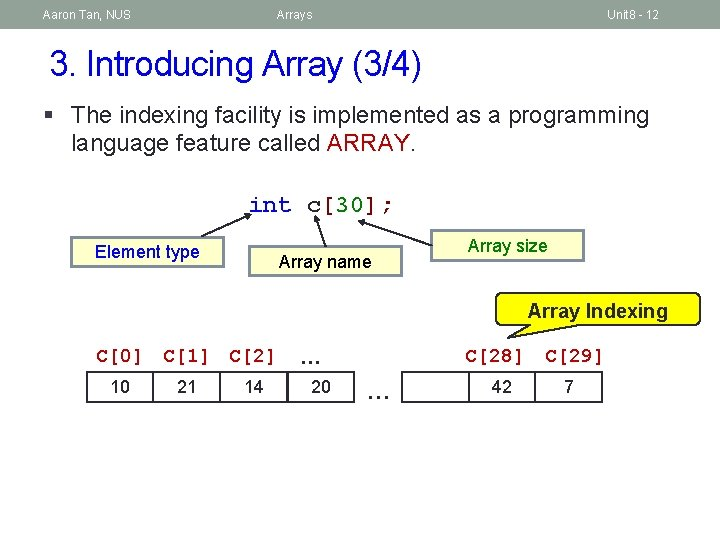Aaron Tan, NUS Arrays Unit 8 - 12 3. Introducing Array (3/4) § The indexing facility is implemented as a programming language feature called ARRAY. int c; Element type Array name Array size Array Indexing C C C 10 21 14 C … 20 … 42 C 7Aaron Tan, NUS Arrays Unit 8 - 13 3. Introducing Array (4/4) Pseudo-code for Vote Counting: Let C be an array such that Ci holds the vote count of Candidate i for each i such that 0 ≤ i < Number_of_candidates Ci 0 ; while there is a vote to be counted vote read a vote if 1 ≤ vote ≤ Number_of_candidate then j vote – 1 Cj + 1Aaron Tan, NUS Arrays Unit 8 - 14 3. 1 Array Declaration: Syntax T arrname [ E ] § § arrname is name/identifier of array (same way you would name a variable) T is a data type (e. g. , int, double, char, …) E is an integer constant expression with a positive value Examples: int arr; // size of arr is 10 #define M 5 #define N 10 double foo[M*N+8]; // size of foo is 58 int i; float bar[i]; // variable-length array Not encouraged to use variable-length arrays. Not supported by ISO C 90 standard. gcc –pedantic will generate warning. For problem using arrays, we will state the maximum number of elements so there is no need for variable-length arrays.Aaron Tan, NUS Arrays Unit 8 - 15 3. 2 Array Variable (1/4) § In an array of type T, each element is a type T variable. Unit 8_Vote. Count. Array. c #define NUM_VOTES 1000 #define NUM_CANDIDATES 30 int main(void) { int i, vote, cand[NUM_CANDIDATES]; for (i = 0; i < NUM_CANDIDATES; i++) // initialize array cand[i] = 0; printf("Enter votes: n"); for (i = 0; i < NUM_VOTES; i++) { scanf("%d", &vote); What is cand[vote-1]++; %%? } for (i = 0; i < NUM_CANDIDATES; i++) { printf("candidate %d: total %d, %. 2 f%%n", i+1, cand[i], (cand[i] * 100. 0)/NUM_VOTES); Why 100. 0 and } not 100? return 0; }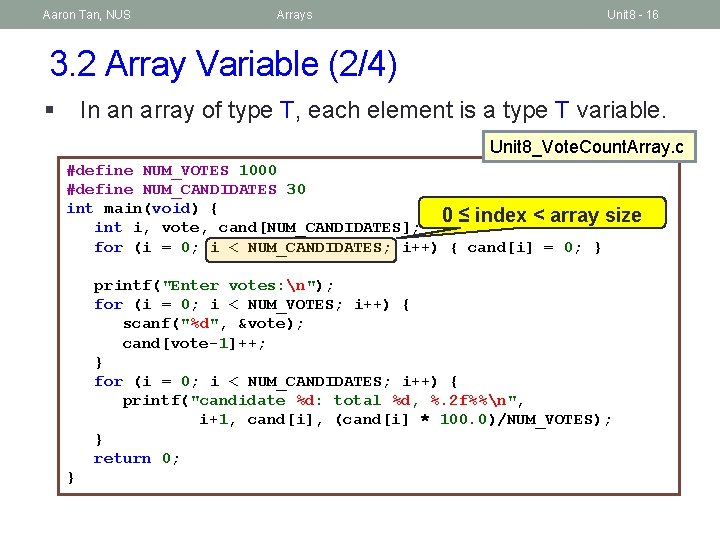Aaron Tan, NUS Arrays Unit 8 - 16 3. 2 Array Variable (2/4) § In an array of type T, each element is a type T variable. Unit 8_Vote. Count. Array. c #define NUM_VOTES 1000 #define NUM_CANDIDATES 30 int main(void) { 0 ≤ index < array size int i, vote, cand[NUM_CANDIDATES]; for (i = 0; i < NUM_CANDIDATES; i++) { cand[i] = 0; } printf("Enter votes: n"); for (i = 0; i < NUM_VOTES; i++) { scanf("%d", &vote); cand[vote-1]++; } for (i = 0; i < NUM_CANDIDATES; i++) { printf("candidate %d: total %d, %. 2 f%%n", i+1, cand[i], (cand[i] * 100. 0)/NUM_VOTES); } return 0; }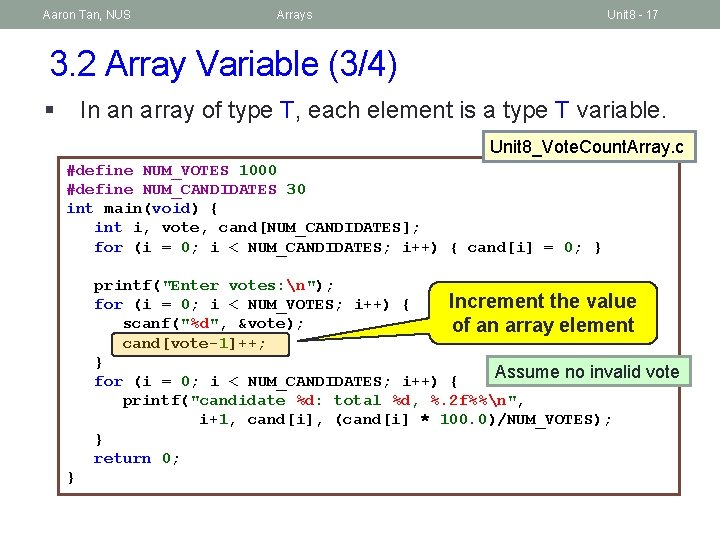Aaron Tan, NUS Arrays Unit 8 - 17 3. 2 Array Variable (3/4) § In an array of type T, each element is a type T variable. Unit 8_Vote. Count. Array. c #define NUM_VOTES 1000 #define NUM_CANDIDATES 30 int main(void) { int i, vote, cand[NUM_CANDIDATES]; for (i = 0; i < NUM_CANDIDATES; i++) { cand[i] = 0; } printf("Enter votes: n"); Increment the value for (i = 0; i < NUM_VOTES; i++) { scanf("%d", &vote); of an array element cand[vote-1]++; } Assume no invalid vote for (i = 0; i < NUM_CANDIDATES; i++) { printf("candidate %d: total %d, %. 2 f%%n", i+1, cand[i], (cand[i] * 100. 0)/NUM_VOTES); } return 0; }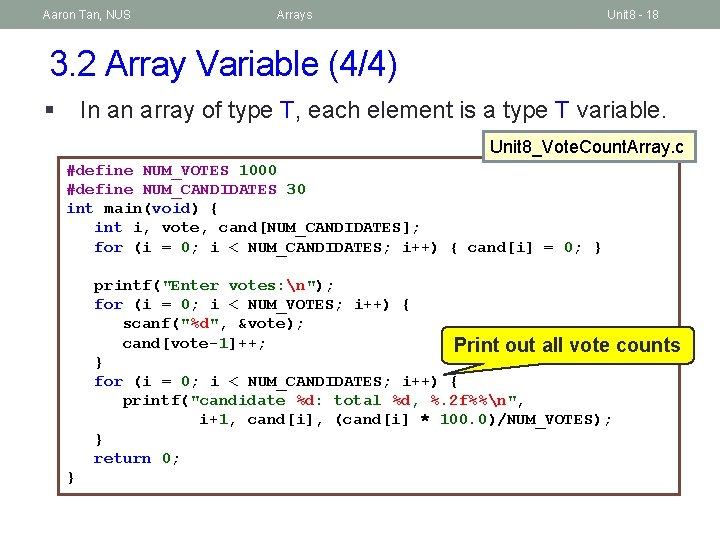Aaron Tan, NUS Arrays Unit 8 - 18 3. 2 Array Variable (4/4) § In an array of type T, each element is a type T variable. Unit 8_Vote. Count. Array. c #define NUM_VOTES 1000 #define NUM_CANDIDATES 30 int main(void) { int i, vote, cand[NUM_CANDIDATES]; for (i = 0; i < NUM_CANDIDATES; i++) { cand[i] = 0; } printf("Enter votes: n"); for (i = 0; i < NUM_VOTES; i++) { scanf("%d", &vote); cand[vote-1]++; Print out all vote counts } for (i = 0; i < NUM_CANDIDATES; i++) { printf("candidate %d: total %d, %. 2 f%%n", i+1, cand[i], (cand[i] * 100. 0)/NUM_VOTES); } return 0; }Aaron Tan, NUS Arrays Unit 8 - 19 3. 3 Array Declarations with Initializers § Array variables can be initialized at the time of declaration. // a=54, a=9, a=10 int a = {54, 9, 10}; // size of b is 3 with b=1, b=2, b=3 int b[] = {1, 2, 3}; // c=17, c=3, c=10, c=0, c=0 int c = {17, 3, 10}; § The following initializations are incorrect: int e = {1, 2, 3}; // warning issued: excess elements int f; f = {8, 23, 12, -3, 6}; // too late to do this; // compilation errorAaron Tan, NUS Arrays Unit 8 - 20 3. 4 Demo #1: Using Array Initializer § Modify the program to use an array initializer. Unit 8_Vote. Count. Array. Ver 2. c #define NUM_VOTES 1000 #define NUM_CANDIDATES 30 int main(void) { int i, vote, cand[NUM_CANDIDATES]; for (i = 0; i < NUM_CANDIDATES; i++) { cand[i] = 0; } int cand[NUM_CANDIDATES] = { 0 }; printf("Enter votes: n"); for (i = 0; i < NUM_VOTES; i++) { scanf("%d", &vote); cand[vote-1]++; } for (i = 0; i < NUM_CANDIDATES; i++) { printf("candidate %d: total %d, %. 2 f%%n", i+1, cand[i], (cand[i] * 100. 0)/NUM_VOTES); } return 0; }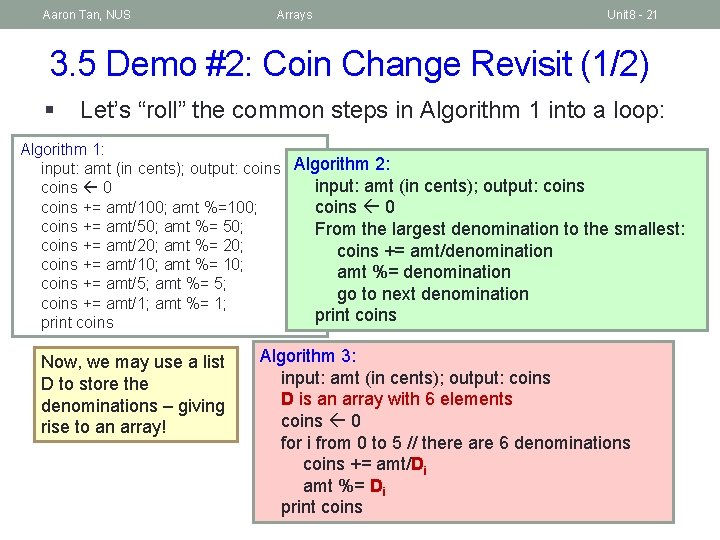Aaron Tan, NUS Arrays Unit 8 - 21 3. 5 Demo #2: Coin Change Revisit (1/2) § Let’s “roll” the common steps in Algorithm 1 into a loop: Algorithm 1: input: amt (in cents); output: coins Algorithm 2: input: amt (in cents); output: coins 0 coins += amt/100; amt %=100; coins 0 coins += amt/50; amt %= 50; From the largest denomination to the smallest: coins += amt/20; amt %= 20; coins += amt/denomination coins += amt/10; amt %= denomination coins += amt/5; amt %= 5; go to next denomination coins += amt/1; amt %= 1; print coins Now, we may use a list D to store the denominations – giving rise to an array! Algorithm 3: input: amt (in cents); output: coins D is an array with 6 elements coins 0 for i from 0 to 5 // there are 6 denominations coins += amt/Di amt %= Di print coins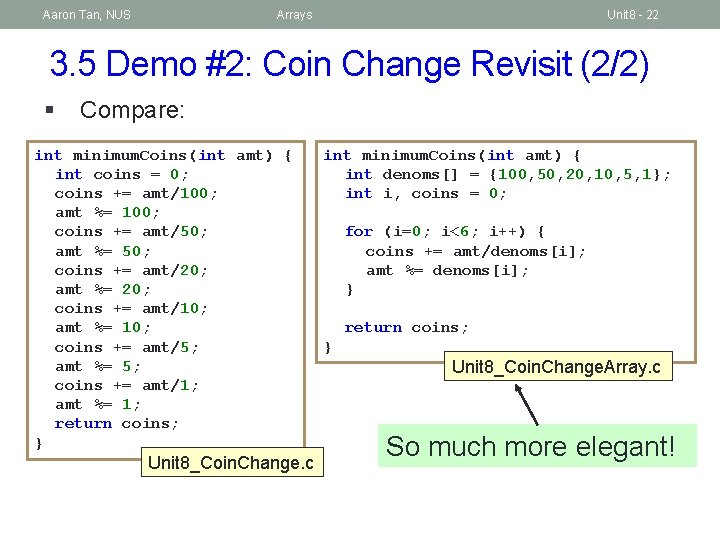Aaron Tan, NUS Arrays Unit 8 - 22 3. 5 Demo #2: Coin Change Revisit (2/2) § Compare: int minimum. Coins(int amt) { int coins = 0; coins += amt/100; amt %= 100; coins += amt/50; amt %= 50; coins += amt/20; amt %= 20; coins += amt/10; amt %= 10; coins += amt/5; amt %= 5; coins += amt/1; amt %= 1; return coins; } Unit 8_Coin. Change. c int minimum. Coins(int amt) { int denoms[] = {100, 50, 20, 10, 5, 1}; int i, coins = 0; for (i=0; i<6; i++) { coins += amt/denoms[i]; amt %= denoms[i]; } return coins; } Unit 8_Coin. Change. Array. c So much more elegant!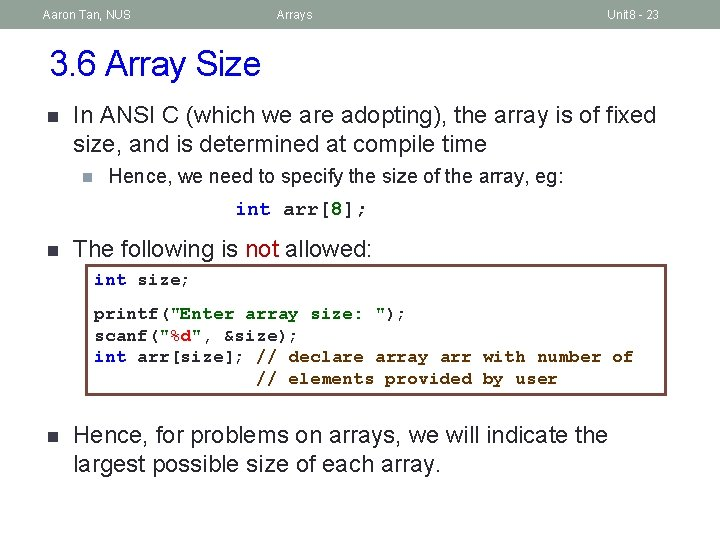Aaron Tan, NUS Arrays Unit 8 - 23 3. 6 Array Size n In ANSI C (which we are adopting), the array is of fixed size, and is determined at compile time n Hence, we need to specify the size of the array, eg: int arr; n The following is not allowed: int size; printf("Enter array size: "); scanf("%d", &size); int arr[size]; // declare array arr with number of // elements provided by user n Hence, for problems on arrays, we will indicate the largest possible size of each array.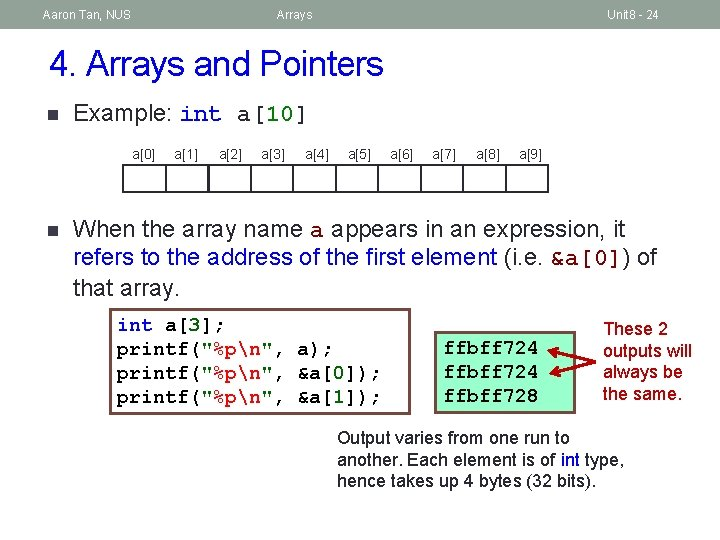Aaron Tan, NUS Arrays Unit 8 - 24 4. Arrays and Pointers n Example: int a a n a a a a a a a a a When the array name a appears in an expression, it refers to the address of the first element (i. e. &a) of that array. int a; printf("%pn", a); printf("%pn", &a); printf("%pn", &a); ffbff 724 ffbff 728 These 2 outputs will always be the same. Output varies from one run to another. Each element is of int type, hence takes up 4 bytes (32 bits).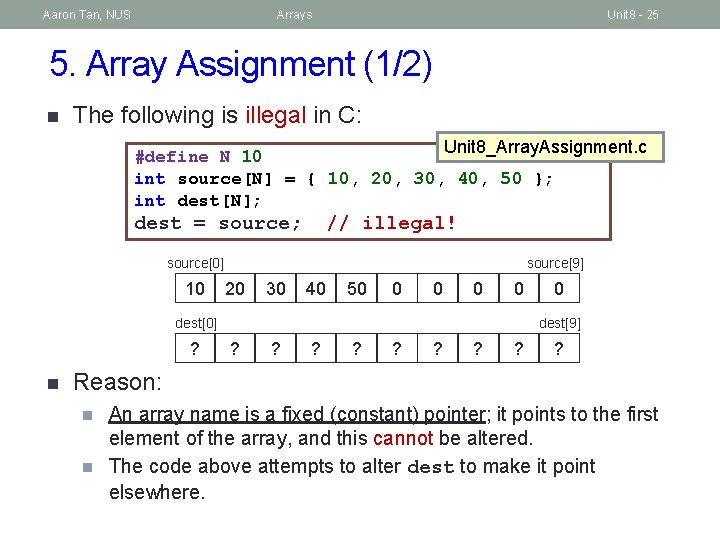Aaron Tan, NUS Arrays Unit 8 - 25 5. Array Assignment (1/2) n The following is illegal in C: Unit 8_Array. Assignment. c #define N 10 int source[N] = { 10, 20, 30, 40, 50 }; int dest[N]; dest = source; // illegal! source 10 source 20 30 40 50 0 0 dest ? n 0 dest ? ? ? Reason: n n An array name is a fixed (constant) pointer; it points to the first element of the array, and this cannot be altered. The code above attempts to alter dest to make it point elsewhere.Aaron Tan, NUS Arrays Unit 8 - 26 5. Array Assignment (2/2) n How to do it properly? Write a loop: Unit 8_Array. Copy. c #define N 10 int source[N] = { 10, 20, 30, 40, 50 }; int dest[N]; int i; for (i = 0; i < N; i++) { dest[i] = source[i]; } source 10 source 20 30 40 50 0 0 dest 10 n 0 dest 20 30 40 50 0 0 (There is another method – use the <string. h> library function memcpy(), but this is outside the scope of CS 1010. )Aaron Tan, NUS Arrays Unit 8 - 27 6. Array Parameters in Functions (1/3) Unit 8_Sum. Array. c int sum. Array(int [], int); // function prototype #include <stdio. h> int main(void) { int foo = {44, 9, 17, 1, -4, 22}; int bar[] = {2, 8, 6}; printf("sum is %dn", sum. Array(foo, 8)); printf("sum is %dn", sum. Array(foo, 3)); printf("sum is %dn", sum. Array(bar, 3)); return 0; } // size of array arr can be unspecified // need an array size parameter int sum. Array(int arr[], int size) { int i, total=0; for (i=0; i<size; i++) total += arr[i]; return total; } sum is 89 sum is 70 sum is 16Aaron Tan, NUS Arrays Unit 8 - 28 6. Array Parameters in Functions (2/3) n Function prototype: n As mentioned before, name of parameters in a function prototype are optional and ignored by the compiler. Hence, both of the following are acceptable and equivalent: int sum. Array(int [], int); int sum. Array(int arr[], int size); n Function header: n n No need to put array size inside [ ]; even if array size is present, compiler just ignores it. Instead, provide the array size through another parameter. int sum. Array(int arr[], int size) {. . . } int sum. Array(int arr, int size) {. . . } Ignored by compiler Actual number of elements you want to process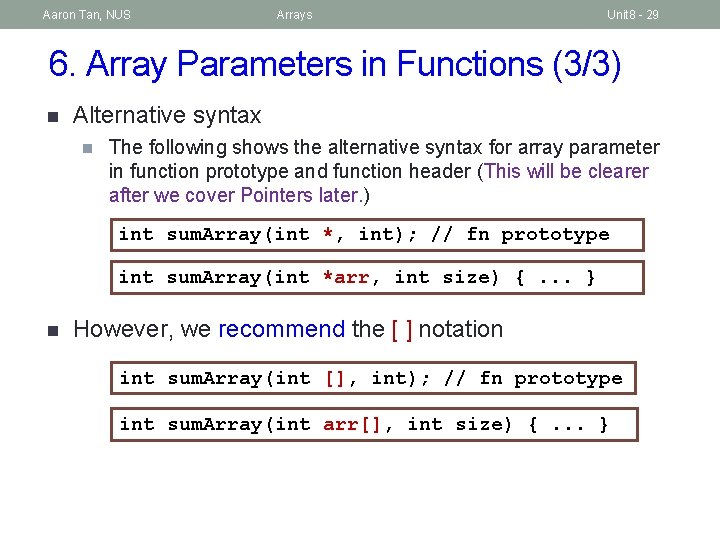Aaron Tan, NUS Arrays Unit 8 - 29 6. Array Parameters in Functions (3/3) n Alternative syntax n The following shows the alternative syntax for array parameter in function prototype and function header (This will be clearer after we cover Pointers later. ) int sum. Array(int *, int); // fn prototype int sum. Array(int *arr, int size) {. . . } n However, we recommend the [ ] notation int sum. Array(int [], int); // fn prototype int sum. Array(int arr[], int size) {. . . }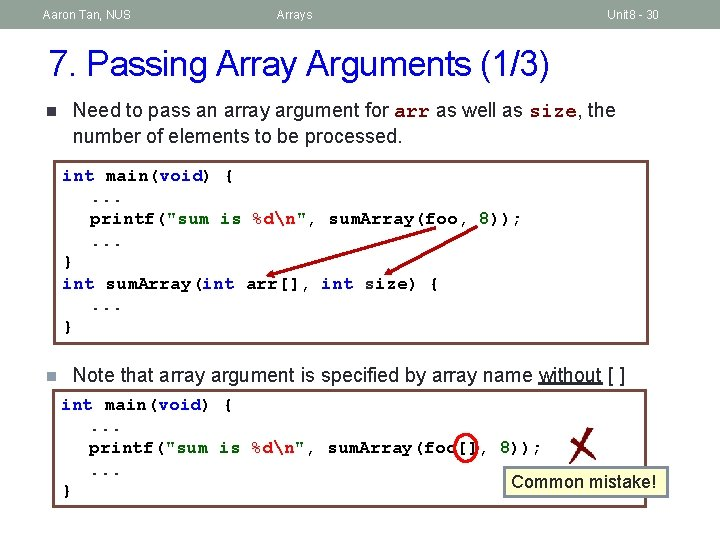Aaron Tan, NUS Arrays Unit 8 - 30 7. Passing Array Arguments (1/3) n Need to pass an array argument for arr as well as size, the number of elements to be processed. int main(void) {. . . printf("sum is %dn", sum. Array(foo, 8)); . . . } int sum. Array(int arr[], int size) {. . . } n Note that array argument is specified by array name without [ ] int main(void) {. . . printf("sum is %dn", sum. Array(foo[], 8)); . . . Common mistake! }Aaron Tan, NUS Arrays Unit 8 - 31 7. Passing Array Arguments (2/3) n Caution! n When passing the value into the parameter representing the number of array elements to be processed, the value must not exceed the actual array size. printf("sum is %dn", sum. Array(foo, 10)); Too big! And compiler won’t be able to detect such “error”! May get “Segmentation Fault (core dumped)” when you run the program!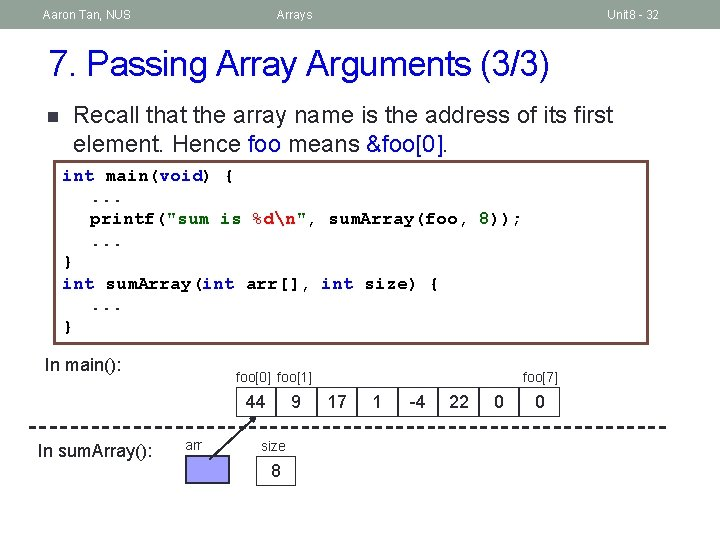Aaron Tan, NUS Arrays Unit 8 - 32 7. Passing Array Arguments (3/3) n Recall that the array name is the address of its first element. Hence foo means &foo. int main(void) {. . . printf("sum is %dn", sum. Array(foo, 8)); . . . } int sum. Array(int arr[], int size) {. . . } In main(): foo foo 44 In sum. Array(): arr 9 size 8 foo 17 1 -4 22 0 0Aaron Tan, NUS Arrays Unit 8 - 33 8. Standard I/O Functions for Arrays (1/3) n It might be advisable to write a function to read values into an array, and a function to print values in an array. n Especially so for the latter, as you probably want to use it to check the values of your array elements at different stages of your program. n The following illustrates an array scores of type float. #define SIZE 6 int main(void) { float scores[SIZE]; scan. Array(scores, SIZE); print. Array(scores, SIZE); return 0; } Unit 8_Array. Input. Output. cAaron Tan, NUS Arrays Unit 8 - 34 8. Standard I/O Functions for Arrays (2/3) n Input function: void scan. Array(float arr[], int size) { int i; float value; // You may add a prompt for user here for (i=0; i<size; i++) { scanf("%f", &value); arr[i] = value; } } or void scan. Array(float arr[], int size) { int i; // You may add a prompt for user here for (i=0; i<size; i++) { scanf("%f", &arr[i]); } }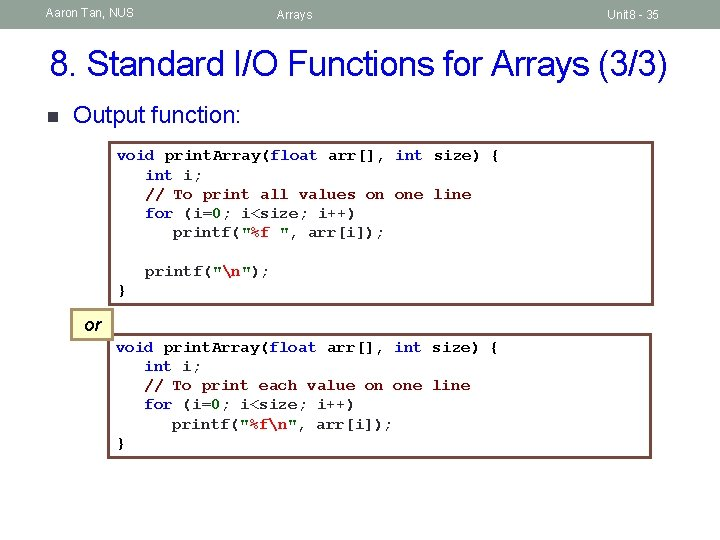Aaron Tan, NUS Arrays Unit 8 - 35 8. Standard I/O Functions for Arrays (3/3) n Output function: void print. Array(float arr[], int size) { int i; // To print all values on one line for (i=0; i<size; i++) printf("%f ", arr[i]); printf("n"); } or void print. Array(float arr[], int size) { int i; // To print each value on one line for (i=0; i<size; i++) printf("%fn", arr[i]); }Aaron Tan, NUS Arrays Unit 8 - 36 9. Modifying Array Arguments (1/2) n Study this program: Unit 8_Modify. Array. Arg. c int main(void) { int foo = {44, 9, 17, 1, -4, 22}; double. Array(foo, 4); print. Array(foo, 8); return 0; } // To double the values of array elements void double. Array(int arr[], int size) { int i; for (i=0; i<size; i++) arr[i] *= 2; } // To print arr void print. Array(int arr[], int size) { int i; for (i=0; i<size; i++) printf("%d ", arr[i]); printf("n"); }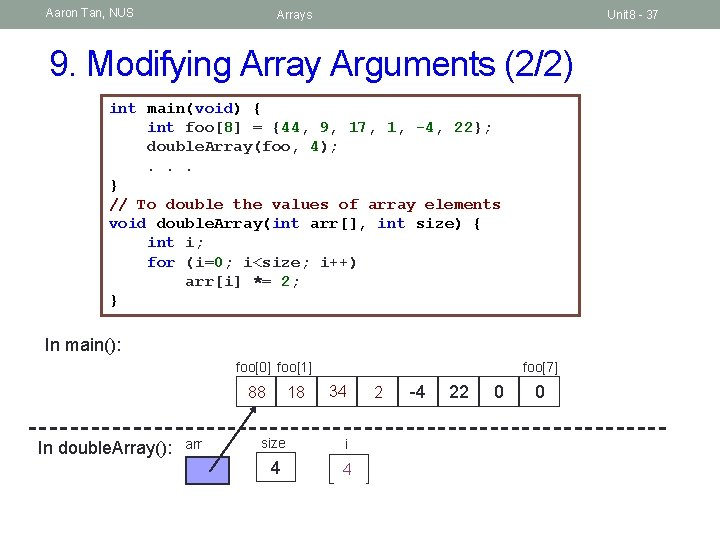Aaron Tan, NUS Arrays Unit 8 - 37 9. Modifying Array Arguments (2/2) int main(void) { int foo = {44, 9, 17, 1, -4, 22}; double. Array(foo, 4); . . . } // To double the values of array elements void double. Array(int arr[], int size) { int i; for (i=0; i<size; i++) arr[i] *= 2; } In main(): foo foo 44 88 In double. Array(): arr 18 9 foo 34 17 size i 4 0 4 21 ? 3 12 -4 22 0 0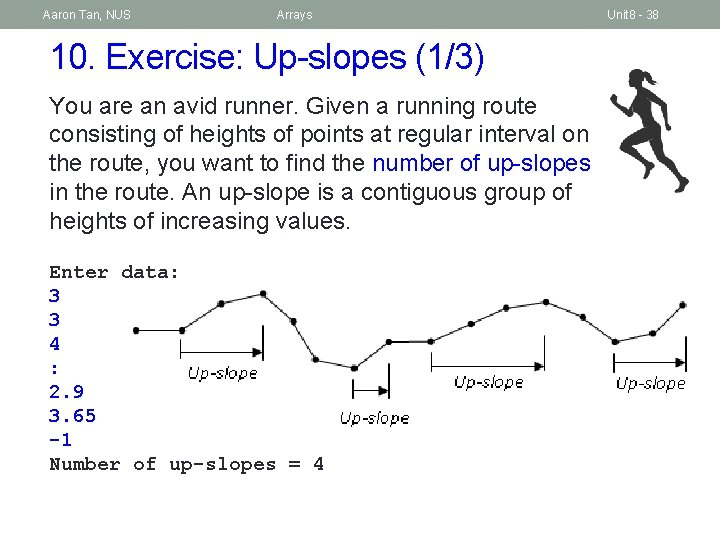Aaron Tan, NUS Arrays 10. Exercise: Up-slopes (1/3) You are an avid runner. Given a running route consisting of heights of points at regular interval on the route, you want to find the number of up-slopes in the route. An up-slope is a contiguous group of heights of increasing values. Enter data: 3 3 4 : 2. 9 3. 65 -1 Number of up-slopes = 4 Unit 8 - 38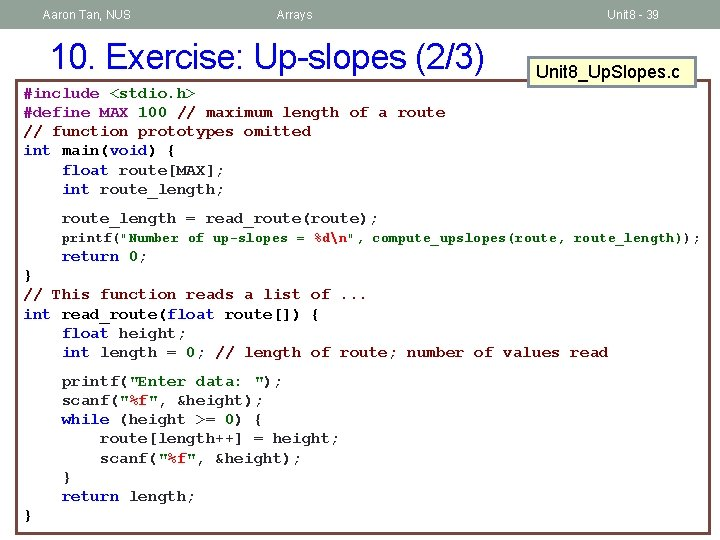Aaron Tan, NUS Arrays 10. Exercise: Up-slopes (2/3) Unit 8 - 39 Unit 8_Up. Slopes. c #include <stdio. h> #define MAX 100 // maximum length of a route // function prototypes omitted int main(void) { float route[MAX]; int route_length; route_length = read_route(route); printf("Number of up-slopes = %dn", compute_upslopes(route, route_length)); return 0; } // This function reads a list of. . . int read_route(float route[]) { float height; int length = 0; // length of route; number of values read printf("Enter data: "); scanf("%f", &height); while (height >= 0) { route[length++] = height; scanf("%f", &height); } return length; }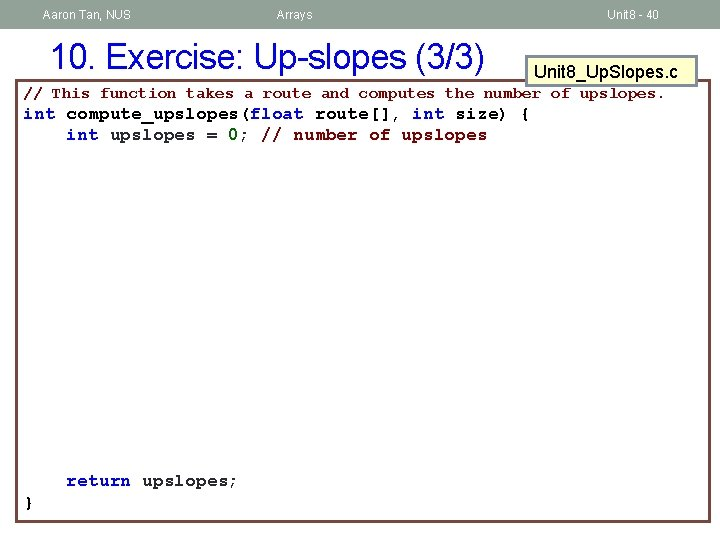Aaron Tan, NUS Arrays 10. Exercise: Up-slopes (3/3) Unit 8 - 40 Unit 8_Up. Slopes. c // This function takes a route and computes the number of upslopes. int compute_upslopes(float route[], int size) { int upslopes = 0; // number of upslopes return upslopes; }Aaron Tan, NUS Arrays Summary n In this unit, you have learned about n Declaring array variables n Using array initializers n Relationship between array and pointer n How to pass an array into a function Unit 8 - 41Aaron Tan, NUS Arrays End of File Unit 8 - 42